# Quantitative Aptitude Quiz for IBPS 2020 Mains Exams- 10th December

Directions (1-5): Given below line graph which shows number of days taken by six persons A, B, C, D, E and F to complete a work individually. Give answer of the questions according to graph and data given in questions —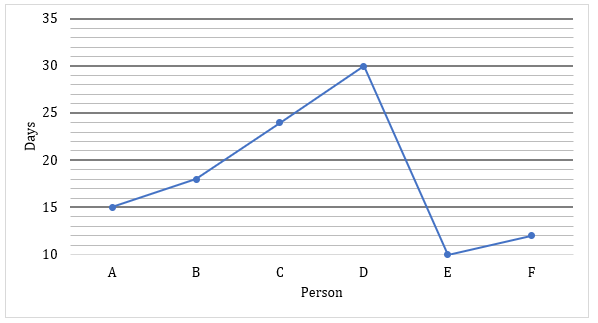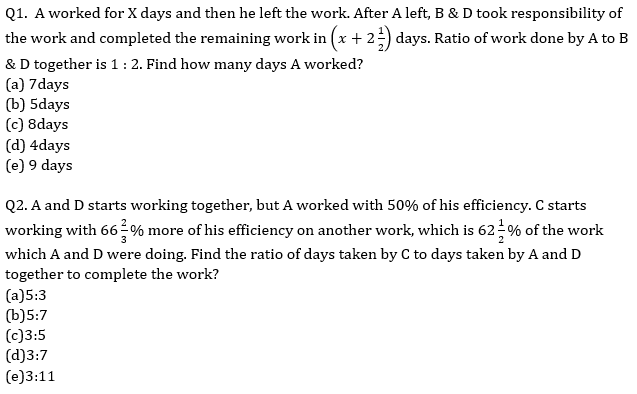Q3. D started the work and left it after working for X days and then C joined the work and worked for Y days. After C left, E completed the remaining work in 3 days. Find number of days for which D and C worked individually, given that value of Y is 200% of value of X?
(a) 6 days & 12 days
(b) 8 days & 16 days
(c) 7 days & 14 days
(d) 9 days & 18 days
(e) 5 days & 10 days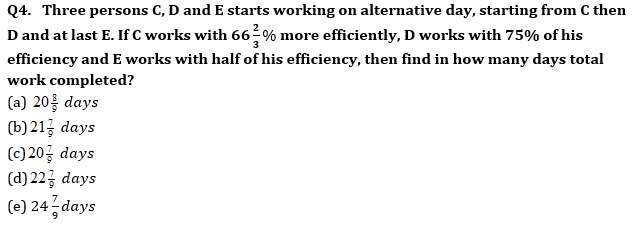Q5. Three persons B, D and F starts working together. If B works with 75% of his efficiency and D worked 25% more efficiently. All three get 27180 Rs. as total wages. Find the individually wage of all according to work done by them (in Rs.)?
(a) 6795, 6795 & 13590
(b)7695, 7695, &13590
(c)6595, 6595, & 14590
(d) 7965, 6795, & 12590
(e) None of these

Directions (6-10): Given below is the table which shows the ratio of distance travelled on Monday to Tuesday by five persons and ratio of speed of these persons on Monday to Tuesday. There is also a line graph which shows the time taken by these persons to cover the given distance on Tuesday with the speed of Tuesday.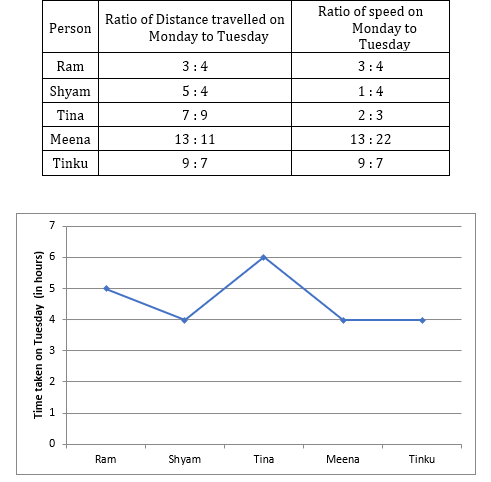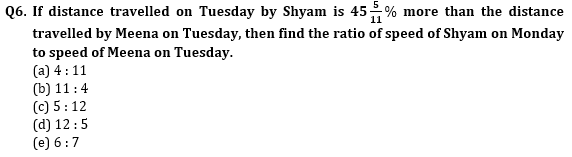Q7. If Ram and Tinku are 600 km apart and they start moving towards each other with the speed of Tuesday, then they meet after 4 hours. If Ram covered 300 km on Monday then find the distance covered by Tinku on Monday.
(a) 2700 km
(b) 300 km
(c) 360 km
(d) 450 km
(e) 500 km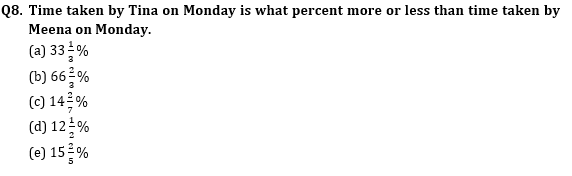Q9. What is the ratio of distance covered by Ram and Shyam on Monday if difference between Total distance covered by Ram on Monday and Tuesday together and Shyam on Monday and Tuesday together is 740 km and speed of Ram of Tuesday is 20 km/hr more than speed of Monday.
(a) 9 : 11
(b) 7 : 8
(c) 3 : 7
(d) 5 : 3
(e) 3 : 8

Q10. If Shyam had travelled 800 km on Monday and Tinku had covered 360 km on Monday, then find the ratio of speed of Shyam on Monday to speed of Tinku on Monday.
(a) 9 : 4
(b) 4 : 9
(c) 3 : 2
(d) 2 : 3
(e) 1 : 2

Directions (11-15): Read the given information carefully and answer the following questions.

Arun & Veer invested Rs. 60,000 and Rs. 90,000 respectively. Arun invested for 15 months while Veer invested for 8 months and the difference between profit share of Arun and Veer is Rs.10000. Arun invested his profit share at 20% p.a on CI for 1.5 years and he bought a bike from the amount he received. Veer bought two laptops L₁ & L₂ (both laptops have equal price) from the amount which he received after investing his profit share at 15% p.a on SI for 2 years. Veer sold L₁ & L₂ at 10% and 15% profit respectively while Arun sold his bike at 5% loss.

Q11. Veer invested money (which he gets from the profit of that two laptops) in a scheme at the rate of 10% p.a on simple interest for 4 years. What is the interest he received?
(a) Rs. 2800
(b) Rs. 2600
(c) Rs. 2500
(d) Rs. 2750
(e) Rs. 3000

Q12. If the price of Bike depreciates 15% every year, then what will be the price of bike after 2 years?
(a) Rs. 47,685
(b) Rs. 49,675
(c) Rs. 48,025
(d) Rs. 47,515
(e) Rs. 48,195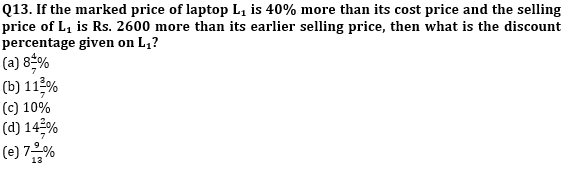Q14. The total amount received by Arun on selling that bike and the total amount received by veer on selling both the laptops together is how much more or less than the 108% of total cost price of all the three items together?
(a) Rs. 6040
(b) Rs. 6180
(c) Rs. 6240
(d) Rs. 6340
(e) Rs. 6380

Q15. In the above passage, if the difference between profit share of Arun and Veer is Rs. 11000 instead of Rs. 10000, then what will be the change in the net profit earned on all the three items together? (Rest all information remains same)
(a) Rs. 120
(b) Rs. 320
(c) Rs. 220
(d) Rs. 520
(e) Rs. 420

Practice More Questions of Quantitative Aptitude for Competitive Exams:

###### Mains Quiz Study Plan for IBPS Exams 2020

Solutions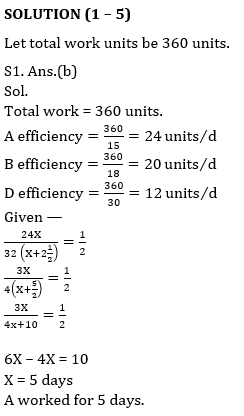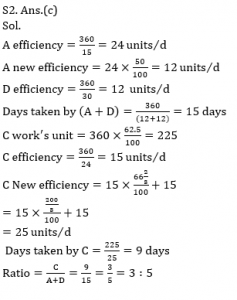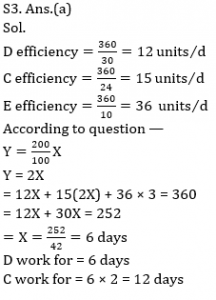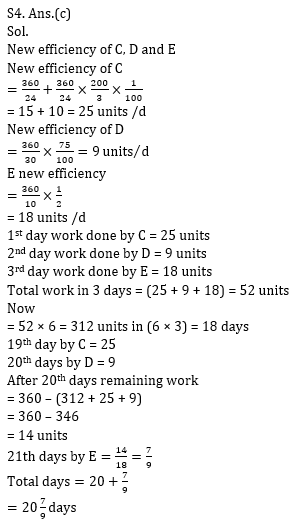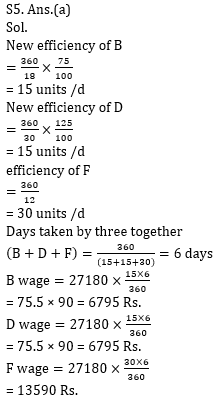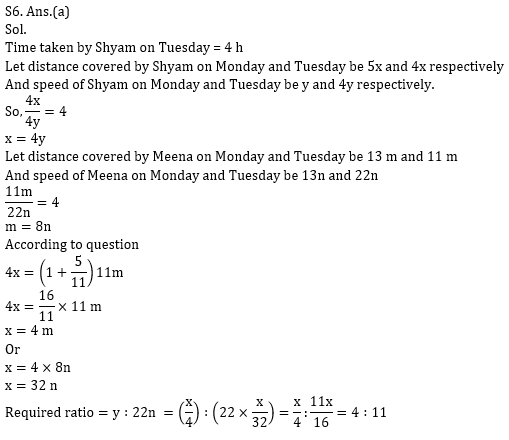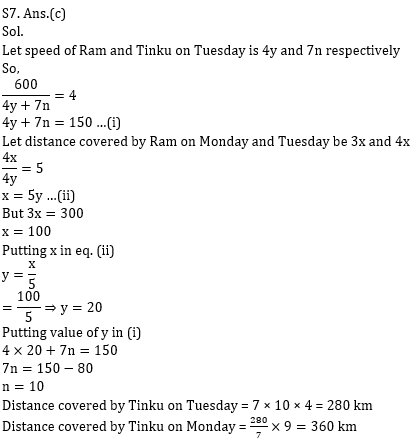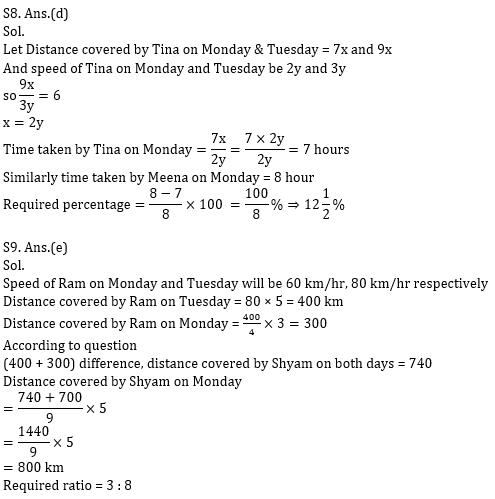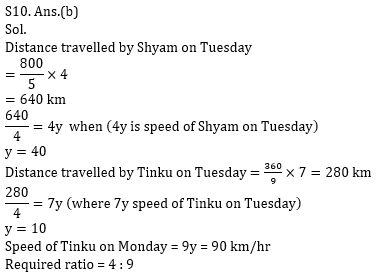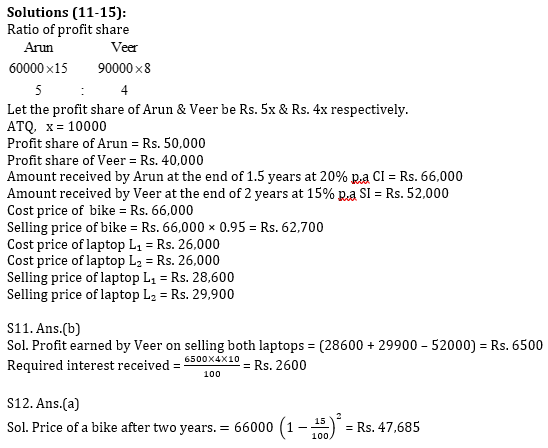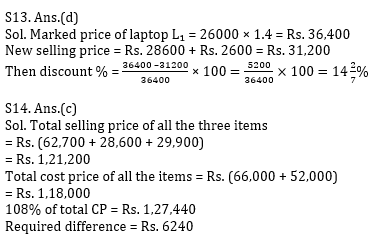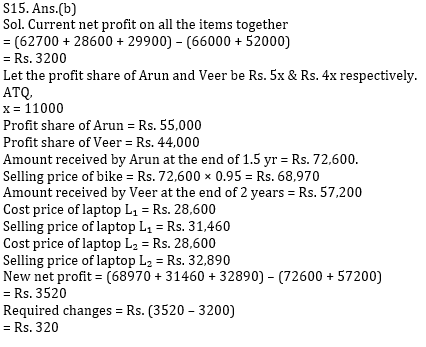Practice with Crash Course and Online Test Series for IBPS Clerk Prelims: